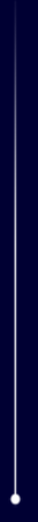Important notice about the STEREO redirects

## Explanation of Coordinate Systems

The coordinate systems used on the "Where is STEREO?" page follow the definitions given in the following references

• Fränz, M. and Harper, D. (2002). Heliospheric coordinate systems. Planetary and Space Science, 50, 217-239.
• Hapgood, M. A. (1992). Space physics coordinate transformation: A user guide. Planetary and Space Science, 40, 711-717.
• Russell, C. T. (1971). Geophysical coordinate transformations. Cosmic Electrodynamics, 2, 184-196.
Below, each coordinate system is summarized.

Note that the various ecliptic coordinate systems can differ in how precession is handled. Ecliptic coordinates can either be referenced to the J2000 epoch, or precessed to the epoch of date. We follow the conventions of Fränz and Harper (2002) in deciding which to apply for each coordinate system. In the table below, the notation "J2000" means that the coordinates are referenced to the J2000 epoch, while "D" means that the coordinates were precessed to the mean ecliptic of date.

For each coordinate system, two axes are given. The third axis in each case completes the right-handed system.

Geocentric coordinate systems
GEIGeocentric Equatorial Inertial X=First point of Aries
Z=Geographic north pole
J2000
GEOGeographic X=Intersection of Greenwich meridian and geographic equator
Z=Geographic north pole

GSEGeocentric Solar Ecliptic X=Earth-Sun line
Z=Ecliptic north pole
D
Heliocentric coordinate systems
HCIHeliocentric Inertial Z=Solar rotational axis
X=Solar ascending node on ecliptic
J2000
HAEHeliocentric Aries Ecliptic X=First point of Aries
Z=Ecliptic north pole
J2000
HEEHeliocentric Earth Ecliptic X=Sun-Earth line
Z=Ecliptic north pole
D
HEEQHeliocentric Earth Equatorial Z=Solar rotation axis
X=intersection of solar equator and solar central meridian as seen from Earth

The calculations are based on the JPL/NAIF SPICE package, using IDL software which is available as part of the Solar Software Library. A user's guide is available in both PDF and Postscript formats.

Roll angles for the two spacecraft are defined such that the nominal orientation of each spacecraft is close to zero degrees. This is done by subtracting 180 degrees from the Behind values reported by the spacecraft. After the two spacecraft pass behind the Sun in 2015, the definition of "nominal" orientation will need to be changed--this is not currently handled by the software.

Last Revised: Thursday, 22-Apr-2010 21:10:37 UTC
Responsible NASA Official: